Processing ......FreeComputerBooks.com Links to Free Computer, Mathematics, Technical Books all over the World

Fundamentals of Cryptology: A Professional Reference and Interactive Tutorial
🌠 Top Free Data Science Books - 100% Free or Open Source!
• Title Fundamentals of Cryptology: A Professional Reference and Interactive Tutorial
• Author(s) Henk C.A. Tilborg
• Publisher: Kluwer Academic Publishers , Boston etc., 2000.
• Hardcover: 512 pages
• eBook: PDF, 2.86 MB
• Language: English
• ISBN-10: 0792386752
• ISBN-13: 978-0792386759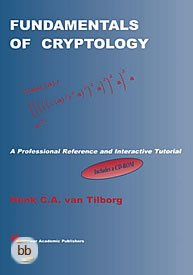Book Description

This is a thoroughly written wonderful book on cryptography! This excellent book is warmly recommended for mathematicians, computer scientists, for professors and students, and, as a reference book, for researchers in the area of cryptography.

The protection of sensitive information against unauthorized access or fraudulent changes has been of prime concern throughout the centuries. Modern communication techniques, using computers connected through networks, make all data even more vulnerable to these threats. In addition, new issues have surfaced that did not exist previously, e.g. adding a signature to an electronic document.

Cryptology addresses the above issues -- it is at the foundation of all information security. The techniques employed to this end have become increasingly mathematical in nature. Fundamentals of Cryptology serves as an introduction to modern cryptographic methods. After a brief survey of classical cryptosystems, it concentrates on three main areas.

First, stream ciphers and block ciphers are discussed. These systems have extremely fast implementations, but sender and receiver must share a secret key. Second, the book presents public key cryptosystems, which make it possible to protect data without a prearranged key. Their security is based on intractable mathematical problems, such as the factorization of large numbers.

The remaining chapters cover a variety of topics, including zero-knowledge proofs, secret sharing schemes and authentication codes. Two appendices explain all mathematical prerequisites in detail: one presents elementary number theory (Euclid's Algorithm, the Chinese Remainder Theorem, quadratic residues, inversion formulas, and continued fractions) and the other introduces finite fields and their algebraic structure.

Fundamentals of Cryptology is an updated and improved version of An Introduction to Cryptology, originally published in 1988. Apart from a revision of the existing material, there are many new sections, and two new chapters on elliptic curves and authentication codes, respectively.

In addition, the book is accompanied by a full text electronic version on CD-ROM as an interactive Mathematica manuscript. Fundamentals of Cryptology will be of interest to computer scientists, mathematicians, and researchers, students, and practitioners in the area of cryptography.

• N/A
Reviews, Ratings, and Recommendations: Related Book Categories: Read and Download Links:Similar Books:
•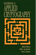Handbook of Applied Cryptography (Alfred J. Menezes, et al)

This book is an incredible achievement. If you want to check what problems there were with a proposed system, determine how the variations on a particular algorithm developed, see what research preceded and followed an idea, go to the Handbook.

•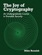The Joy of Cryptography (Mike Rosulek)

The definitive introduction to all major areas of cryptography: message security, key negotiation, and key management, but in a way that is more accessible than what is "traditional" in crypto. All security definitions are written in a "game-based" style.

•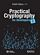Practical Cryptography for Developers (Svetlin Nakov)

A modern practical book about cryptography for developers with code examples, covering core concepts, along with crypto libraries and developer tools, with a lots of code examples in Python and other languages.

•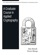A Graduate Course in Applied Cryptography (Dan Boneh, et al)

This book is about mathematical modeling and proofs to show that a particular cryptosystem satisfies the security properties attributed to it: constructing practical cryptosystems for which we can argue security under plausible assumptions.

• Similar Books:
•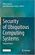Security of Ubiquitous Computing Systems (Gildas Avoine, et al)

It is to improve and adapt existent cryptanalysis methodologies and tools to the ubiquitous computing framework that lies along four axes: cryptographic models, cryptanalysis of building blocks, security engineering, and security assessment.

•Handbook of Applied Cryptography (Alfred J. Menezes, et al)

This book is an incredible achievement. If you want to check what problems there were with a proposed system, determine how the variations on a particular algorithm developed, see what research preceded and followed an idea, go to the Handbook.

•The Joy of Cryptography (Mike Rosulek)

The definitive introduction to all major areas of cryptography: message security, key negotiation, and key management, but in a way that is more accessible than what is "traditional" in crypto. All security definitions are written in a "game-based" style.

•Practical Cryptography for Developers (Svetlin Nakov)

A modern practical book about cryptography for developers with code examples, covering core concepts, along with crypto libraries and developer tools, with a lots of code examples in Python and other languages.

•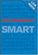Cryptography: An Introduction, 3rd Edition (Nigel Smart)

This book provides the rigorous detail required for advanced cryptographic studies, yet approaches the subject matter in an accessible style in order to gently guide new students through difficult mathematical topics.

•A Graduate Course in Applied Cryptography (Dan Boneh, et al)

This book is about mathematical modeling and proofs to show that a particular cryptosystem satisfies the security properties attributed to it: constructing practical cryptosystems for which we can argue security under plausible assumptions.

•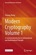Modern Cryptography Volume 1: A Classical Introduction

This book systematically explores the statistical characteristics of cryptographic systems, the computational complexity theory of cryptographic algorithms and the mathematical principles behind various encryption and decryption algorithms.

•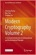Modern Cryptography Volume 2: Post-Quantum Cryptography

This open access book covers the most cutting-edge and hot research topics and fields of post-quantum cryptography. The main purpose of this book is to focus on the computational complexity theory of lattice ciphers.

•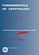Fundamentals of Cryptology: A Professional Reference (H. Tilborg)

This is a thoroughly written wonderful book on cryptography! This excellent book is warmly recommended for mathematicians, computer scientists, for professors and students, and, as a reference book, for researchers in the area of cryptography.

•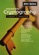Introduction to Modern Cryptography (Mihir Bellare, et al.)

This book introduces the core principles of modern cryptography, with an emphasis on formal definitions, clear assumptions, and rigorous proofs of security. It also presents design principles for widely used stream ciphers and block ciphers.

•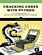Cracking Codes with Python: Building and Breaking Ciphers

Learn how to program in Python while making and breaking ciphers - algorithms used to create and send secret messages! You'll begin with simple programs for the reverse and Caesar ciphers and then work your way up to public key cryptography, etc.

•Cryptography: An Introduction, 3rd Edition (Nigel Smart)

This book provides the rigorous detail required for advanced cryptographic studies, yet approaches the subject matter in an accessible style in order to gently guide new students through difficult mathematical topics.

•Introduction to Modern Cryptography (Mihir Bellare, et al.)

This book introduces the core principles of modern cryptography, with an emphasis on formal definitions, clear assumptions, and rigorous proofs of security. It also presents design principles for widely used stream ciphers and block ciphers.

Book Categories
 :All CategoriesTop Free BooksRecent BooksMiscellaneous BooksComputer EngineeringComputer LanguagesComputer ScienceData Science/DatabasesJava and Java EE (J2EE)Linux and UnixMathematicsMicrosoft and .NETMobile ComputingNetworking and CommunicationsSoftware EngineeringSpecial TopicsWeb Programming
Other Categories